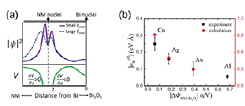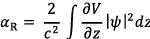# ISSP - The institute for Solid State PhysicsFont Size: (S) / (M) / (L)

- Activity Report 2017 -

Otani Group

Clear Variation of Spin Splitting by Changing Electron Distribution at Non-magnetic Metal/Bi2O3 InterfacesFig. 1. (a) Rashba spin splitting at NM/Bi2O3 interface. (b) Schematic of spin-to-charge conversion at the NM/Bi2O3 interface. A spin current is injected from the Py layer into the NM/Bi2O3 interface, and then converted to the charge current via the inverse Edelstein effect.Fig. 2. (a) An asymmetry distribution of |Ψ|2 generated by interfacial electric field. Purple line and blue line show the |Ψ|2 under smaller and larger electric field, respectively. Green line show electrostatic potential V. (b) Absolute value of αR estimated by the experiment and the calculation in different NM/Bi2O3 interfaces as a function of |ΔΦNM-Bi2O3|.

Edelstein effect, the spin-to-charge current interconversion at interfaces , has attracted much attention. An efficient interconversion at Cu/Bismuth oxide (Bi2O3) interface has been discovered [2, 3], which is attributed to a large Rashba splitting in the electronic state at the metal/oxide interface (see Fig. 1 (1)). However, the guiding principle of designing the metal/oxide interface with large Rashba splitting for the efficient conversion is missing. In this study, we report strong non-magnetic (NM) material dependence of spin splitting at NM/Bi2O3 interface and discuss the origin of the NM dependence with first-principles calculations.

We employed spin pumping technique to inject spin current into the NM/Bi2O3 (NM = Al, Cu, Ag, Au) interface. The injected spin current is converted to charge current by the inverse Edelstein effect (spin-to-charge conversion at the interface, see Fig. 1 (b)). We evaluated the conversion coefficient and the Rashba parameter αR which determines the splitting in momentum between spin-up and spin-down electrons. The evaluated αR are −0.055, −0.25, +0.15 and −0.09 eV·Å for NM = Al, Cu, Ag and Au, respectively.

The Rashba parameter αR can be described as,,　　　　　 (1)

where c, ∂V/∂z and |Ψ|2 are the speed of light, potential gradient and electron density distribution, respectively. Schematic illustration of V and |Ψ|2 at NM/Bi2O3 interfaces based on our ab-initio calculation is shown in Fig. 2 (a). |Ψ|2 can be modulated by the out-of-plane electric field Einter at the interface. Note that Einter originates from work function difference ΔΦNM-Bi2O3 between NM and Bi2O3. The asymmetry feature of |Ψ|2 which depends on Einter and ΔΦNM-Bi2O3 should be important for the magnitude and the sign of αR.

Figure. 2(b) shows |αR| estimated by experiment and calculation in different NM/Bi2O3 as function of |ΔΦNM-Bi2O3|. |αR| decreases as |ΔΦNM-Bi2O3| increases and the trend of calculated |αR| is in good agreement with the experiment. This trend can be explained as follows (see Fig. 2 (a)). |Ψ|2 is strongly localized near NM nuclei when Einter is small, while |Ψ|2 could be shifted from nuclei and delocalized when Einter is large. The steepest ∂V/∂z is located near NM nuclei. Thus, larger Einter (larger |ΔΦNM-Bi2O3|) gives smaller integral in Eq. (1). The opposite sign of αR between Ag/Bi2O3 and other interfaces may be explained by different sign of ΔΦNM-Bi2O3 at Ag/Bi2O3 from others. Assuming that Ag/Bi2O3 and Cu/Bi2O3 interfaces have similar hybridization state, the opposite direction of Einter may shift the |Ψ|2 to different side of NM or Bi2O3 and cause the sign change of the integral.

We found that the asymmetry feature of |Ψ|2 which can be modulated by ΔΦNM-Bi2O3 results in strong NM dependence on αR. Our study suggests that control of interfacial electron distribution by changing the difference in work function across the interface may be an effective way to tune the magnitude and sign of Rashba splitting and the spin-to-charge current interconversion at interface.

References

•  J. C. R. Sánchez et al., Nat. Commun. 4, 2944 (2013).
•  S. Karube, K. Kondou, and Y. C. Otani, Appl. Phys. Express 9, 033001(2016).
•  J. Kim, Y. T. Chen, S. Karube, S. Takahashi, K. Kondou, G. Tatara, and Y. Otani, Phys. Rev. B 96, 140409(R) (2017).

Authers

• H. Isshiki and Y. Otani